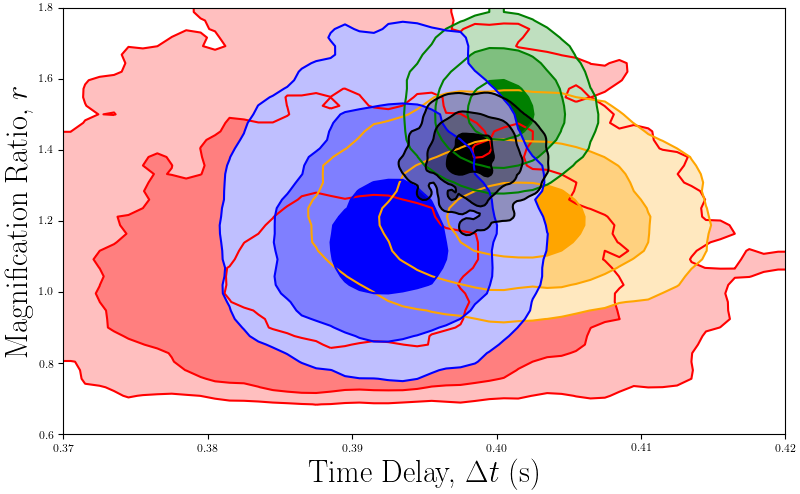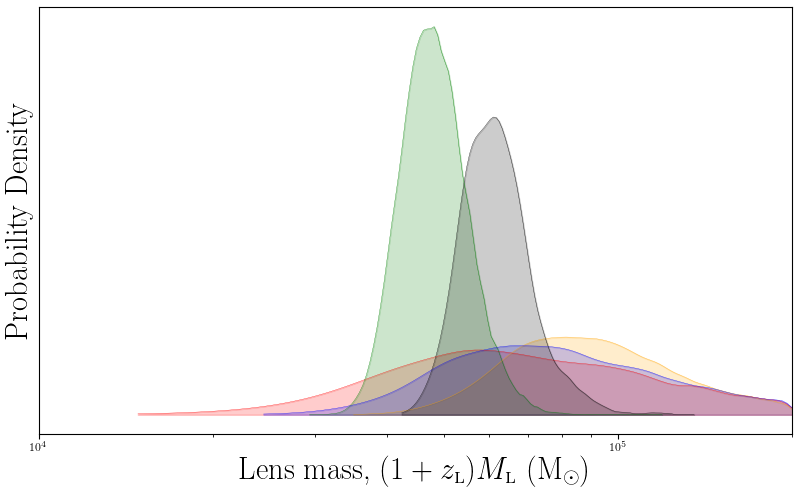# GRB 950830 code walkthrough¶

## Walkthrough¶

We first attempt to fit all possible combinations of pulse types. We find that in the strong majority of cases, a lensing model is preferred over a model of two individual pulses.

Imports:

import numpy as np

from PyGRB.main.fitpulse import PulseFitter
from PyGRB.backend.makemodels import create_model_from_key


Set up the PulseFitter object:

sampler  = 'dynesty'
nSamples = 2000
GRB = PulseFitter(3770, times = (-.1, 1),
datatype = 'tte', nSamples = nSamples, sampler = sampler,
priors_pulse_start = -.1, priors_pulse_end = 0.6,
priors_td_lo = 0,  priors_td_hi = 0.5)


Note that in some rare instances the code will choose to fit a small residual quite late to the noise, and the ‘gravitationally lens’ that noise. So in the case where one is fitting a lens model, a better choice of start time priors might be priors_pulse_end = 0.3.

Set up the models to fit to the light-curve. lens_keys are a single pulse, which is then ‘gravitationally lensed’ – the light-curve is duplicated at the time delay and rescaled by the magnification ratio. null_keys are two pulses.

lens_keys = ['FL', 'FsL', 'XL', 'XsL']
null_keys = ['FF', 'FsFs', 'XX', 'XsXs']
keys = lens_keys + null_keys

model_dict = {}
for key in keys:
model_dict[key] = create_model_from_key(key)
models = [model for key, model in model_dict.items()]


Then run the model fitting code with

for model in models:
GRB.main_multi_channel(channels = [0, 1, 2, 3], model = model)


## Parallelisation¶

Parallelisation is done through the use of the indices parameter, which can be used to send one channel of one model to a different CPU in the cluster. The difference would be changing the last two lines of code:

# 8 models x 4 channels = 32 separate nested sampling runs.
# This can be cheaply parallelised by sending each run to a different CPU
lens_keys = ['FL', 'FsL', 'XL', 'XsL']
null_keys = ['FF', 'FsFs', 'XX', 'XsXs']
keys = lens_keys + null_keys

model_dict = {}
for key in keys:
model_dict[key] = create_model_from_key(key)
models = [model for key, model in model_dict.items()]

indices = np.arange(32)
GRB._split_array_job_to_4_channels(models = models, indices = indices, channels = [0, 1, 2, 3])


After several days on a HPC, hopefully all the jobs will have finished. The more parameters, the longer the job will take. The ‘FsFs’ and ‘XsXs’ models have 18 and 20 parameters each, and may take up to a week depending on the chosen nSamples (and other sampler keyword arguments). Higher level analysis can be done with the get_evidence_from_models() method:

GRB.get_evidence_from_models(model_dict = model_dict)


To create plots of the gravitational lensing parameters, use the lens_calc method:

for model in models:
lens_bounds = [(0.37, 0.42), (0.60, 1.8)]
GRB.lens_calc(model = model, lens_bounds = lens_bounds)


which will create the following two plotsBATSE trigger 3770 magnification ratio and time delay posterior histogram.

The magnification ratios and time delays are concordant, as one would expect from a gravitational lensing event.BATSE trigger 3770 lens mass posterior histogram.

The inferred lens mass suggests an intermediate mass black hole as the gravitational deflector.